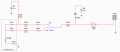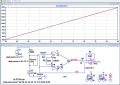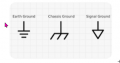# Calculate PT100 resistance value from attached circuit

#### Vindhyachal Takniki

Joined Nov 3, 2014
594
1. Attached is one analog circuit, whose output I can measure.
2. Its three wire PT100 whose resitance I have to measure. RL1=RL2=RL3 is lead wire resistance.
3. Rs1=Rs2 we can take which is resistance of anlog mux displacyed here as switch for simplicity.
4. Output opamp is conencted with gain of 100. After which I can measure voltage by MCU.
5. I can measure two voltage:
a) when switch is top position
b) when switch is at botton position

6. Now who to caluclate PT100 resistance from this?
I tried using thevnin equivalent diagrams but they become very complex with lots of variables.
Need to implement it in MCU.#### ericgibbs

Joined Jan 29, 2010
18,212
hi VT,
Who designed that circuit.?

E

#### Vindhyachal Takniki

Joined Nov 3, 2014
594
THis is someone else design, cannot change.
I have access of circuit on MCU side

#### ericgibbs

Joined Jan 29, 2010
18,212

Last edited:

#### MisterBill2

Joined Jan 23, 2018
16,465
First of all, if there is any requirement fir an accurate indication of the resistance of the RTD, NONE of the connections will include "ground", which is a none-constant value along with unknown random voltages. Thus the circuit as shown is rather inaccurate.

The principle of an RTD system is to pass an accurately controlled current through the "100 ohm"resistor and measure the exact voltage across the resistor. To do that accurately requires FOUR wires, two to supply the current and the other two used to measure the actual voltage across the "100 ohm" resistor..
The three wire scheme is based on the theory that all three wires have the same resistance.. The constant current flows through one wire of the pair, through the "100 ohm" resistor, and returns through the single wire. The voltage between the single wire and the non-current carrying wire Is the sum of the voltage drops of both wires PLUS the voltage drop across the "100 ohm" resistor. SO the voltage drop across the pair is assumed to equal the voltage drop across the two leads, and that value is then subtracted from the measured voltage.
The scheme is fairly accurate, with the penalty being the need to accurately measure two voltages and accurately subtract them. The savings is the cost of one additional wire. That savings has never justified using a 3 terminal scheme in any of the applications of an RTD that I have designed. So that is how the 3 wire system works.

#### Vindhyachal Takniki

Joined Nov 3, 2014
594
1. This analog part is someone else design, cannot change. I have access of circuit on MCU side & can read output of opamp.

2. So considered R32=open due to high value, R31,R33,R34.Rs1,rs2 as wont happen happen much effect on output, as these goes on high input impedance opamp. (thanks to El Tangas for suggestion)

3. Below is equation which comes out, considering R27,Rl1, rl2 & Rl3 in circuit. Rl1=Rl2=Rl3 is taken. Lets call it reduced circuit.
Rp = (60000V2 - 30000V1) / (6.25 - 2.5V1 - 2V1V2)

where Rp= resistance of PT100
V1 & V2 are voltages measured at the input of opamp when switch is at top & bottom

4. Tried simulating circuit on multisim with reduced circuit & matched values with this formula, seems like ok.

5. Is equation can be solved by also considering R31,R33,R34.Rs1,rs2 . I tried but equation starts very complex.
Simulating original circuit, with reduced circuit equation does not work actually.

#### MisterBill2

Joined Jan 23, 2018
16,465
I became lost in your explanation that uses alternative descriptions.
The three wire scheme depends on a perfectly regulated current, and using that perfectly regulated current to determine the resistance of the connecting wires, and measuring the voltage drop in those two wires.
Then, to find the resistance of the loop that includes the PT RTD 100 ohm resistor, it passes exactly the same current through the loop with the 100 ohm PT resistor, and subtracts the voltage drop through the two connecting wires.
The method works fairly well, presuming that the current is perfectly regulated and that all three connecting wires have identical resistance and that the voltage measuring system is perfectly linear. Simulating a constant current regulator by feeding a higher voltage through a higher resistance can be fairly close, and if "fairly close" is good enough then the scheme will be good enough.

#### MisterBill2

Joined Jan 23, 2018
16,465
It is not a benefit to tie a measuring loop to "ground"because it will always reduce the accuracy by adding unknown voltages and currents.
If we read the voltage between wire #1 and wire #2 then we can know the voltage drop in the resistance of wire #1, and we may guess that it is the same as the resistance of wire #3. But because wire #3 is also connected to "ground" we have no knowledge of the current flowing through the R4, nor through the resistance of wire #1, Thus no way to measure the actual resistance of R4.
IF, however, the ground connections are replaced by a single conductor between the negative source connection and the end of wire #3, as the simulation assumes, then the function can be as claimed.
The reality is that "grounds" are never isolated in reality, and so voltages and currents related to them are always unknown.
So unless the two ground connections shown in the circuit of post #4 are a single point connection it is impossible for the circuit to be assured of providing an actually accurate reading.

Now to derive the equations to determine the actual resistance of R4, The 100 ohm RTD resistor, we must accurately know the current passing through it. That is defined as "I", and winds up being the same as it passes through the resistors.Current "I" passes through wires 1 and 3 and so develops a voltage of "I" (Rw1+Rw3) that must be subtracted from the voltage "I"(Rw1+Rw3+R4)
We assume that Rw1=Rw3, and by observation Vbc="I"Rw3+"I"R4="I"(Rw3+R4)
Bot how can we know exactly what the current is??? We can make a close guess and be well withing 1% of the correct value.
Using ohms law, I=V/R, with V= 2.5000 volts, and R=(12,000+100+Rw1+Rw3) ohms and call that 12,000+100 ohms=12100 ohms.
Then "I"=(2.5000/12,100) amps =2.5/.121 milliamps=20.66115 mA

Last edited:

#### ericgibbs

Joined Jan 29, 2010
18,212
It is NOT tied to ground in the actual installation!

The TS is trying to derive an Equation for the circuit, please, read his postings before answering.

#### MisterBill2

Joined Jan 23, 2018
16,465
Referencing the circuit in post #1. The voltage difference between the voltage in the switch position shown, minus the voltage with the switch in the opposite position, equals the voltage drop in the tird wire, which must be subtracted from the voltage read with the switch in the lower position. So now the voltage across the sensing resistor can be calculated. ( I am ignoring the contribution due to V8 and R32 )
Then, to calculate the current through the PT resistor, use I=V/R, =2.5V/ (R27+PT100) =2.5v/(12000+100) =2.5v/12100
And thus Rpt100 =V/I=Vmeasured/ 2.5v/12100

#### WBahn

Joined Mar 31, 2012
29,480
Referencing the circuit in post #1. The voltage difference between the voltage in the switch position shown, minus the voltage with the switch in the opposite position, equals the voltage drop in the tird wire, which must be subtracted from the voltage read with the switch in the lower position. So now the voltage across the sensing resistor can be calculated. ( I am ignoring the contribution due to V8 and R32 )
Then, to calculate the current through the PT resistor, use I=V/R, =2.5V/ (R27+PT100) =2.5v/(12000+100) =2.5v/12100
Where is your value of 100 Ω coming from?

You seem to be assuming that the resistance of the PT100 device is 100 Ω, but the whole point is to figure out this resistance since it is unknown.

You also don't seem to be taking into account the presence of RL3.

And thus Rpt100 =V/I=Vmeasured/ 2.5v/12100
What is Vmeasured? There are two voltages that are measured.

#### WBahn

Joined Mar 31, 2012
29,480
1. Attached is one analog circuit, whose output I can measure.
2. Its three wire PT100 whose resitance I have to measure. RL1=RL2=RL3 is lead wire resistance.
3. Rs1=Rs2 we can take which is resistance of anlog mux displacyed here as switch for simplicity.
4. Output opamp is conencted with gain of 100. After which I can measure voltage by MCU.
5. I can measure two voltage:
a) when switch is top position
b) when switch is at botton position

6. Now who to caluclate PT100 resistance from this?
I tried using thevnin equivalent diagrams but they become very complex with lots of variables.
Need to implement it in MCU.

View attachment 274899
Since R32 is much, much greater than any of the other resistances and it is going to a voltage similar to the left hand side, it should have negligible effect.

Since the amplifier is assumed to have very high input impedance, the effect of R33, R34, Rs1, Rs2, and R31 should be negligible.

ASSUMING that your V7 voltage is stable and accurate AND that you have a precision resistor for R27, the current in R27 is given by

Ix = (V7 - Vup)/R27

where Vup is the voltage measured in the upper position.

The voltage drop across RL1 is (Vup - Vdown)

where Vdown in the voltage measured in the lower position.

IF we assume that RL3 = RL1, then the voltage, Vx, across the PT100 is

Vx = Vup - 2*(Vup - Vdown) = 2*Vdown - Vup

This then gives the following for Rx, the resistance of the PT100

Rx = Vx / Ix = (2*Vdown - Vup) / [(V7 - Vup)/R27] = R27 * (2*Vdown - Vup) / (V7 - Vup)

Let's test this out with some values:

Let's say that the temperature is 100 °C, making the PT100 resistance 138.5 Ω, Let's say that the leadwire resistance is 2 Ω.

The total current flowing is

Ix = 2.5V / (12 kΩ + 2 Ω + 138.5 Ω + 2 Ω) = 2.5 V / 12.1425 kΩ = 205.9 uA

This is about 200x the current that might flow in R32, so treating it as negligible is probably a pretty good approximation.

By voltage division,

Vup = 2.5 V * (142.5 Ω / 12.1425 kΩ) = 29.34 mV
Vdown = 2.5 V * (140.5 Ω / 12.1425 kΩ) = 28.93 mV

As you can see, your measurement is going to be looking for voltage differences on the order of just a few millivolts, so you need to be careful about the specs on your amplifier.

What does that then give us for Rx?

Rx = R27 * (2*Vdown - Vup) / (V7 - Vup)
Rx = 12 kΩ * (2*28.93 mV - 29.34 mV) / (2.5 V - 29.34 mV)
Rx = 138.52 Ω

Now, what if our V7 is 5% high without us knowing about it? What resistance will we calculate? How much of an error in temperature will that equate to?

Then, what if our R27 is 1% to high without us knowing about it? Again, what resistance will we calculate? How much of an error in temperature will that equate to?

#### MisterBill2

Joined Jan 23, 2018
16,465
@WBhann. At some reference temperature the value of a 100 ohm PT RTD is 100 ohms. It does not change a lot and so that is a good initial choice.
As for the voltage drop in the single line, The whole concept of the three wire RTD is that the drop in each line is the same, thus measuring the voltages from each switch position gives two values, the difference being equal to the resistance drop in the third wire. That is the whole concept of the three wire scheme. If greater accuracy is required then the choice is to use a four wire RTD and the actual Kelvin connection method. And if such accuracy is required then the expense of a regulated current is reasonable, with the expense of so many precise resistance values no longer required.

#### WBahn

Joined Mar 31, 2012
29,480
@WBhann. At some reference temperature the value of a 100 ohm PT RTD is 100 ohms. It does not change a lot and so that is a good initial choice.
As for the voltage drop in the single line, The whole concept of the three wire RTD is that the drop in each line is the same, thus measuring the voltages from each switch position gives two values, the difference being equal to the resistance drop in the third wire. That is the whole concept of the three wire scheme. If greater accuracy is required then the choice is to use a four wire RTD and the actual Kelvin connection method. And if such accuracy is required then the expense of a regulated current is reasonable, with the expense of so many precise resistance values no longer required.
I went back and read your post more carefully. You didn't define what your Vmeasured was, but I now see that you described it in words as:

Vmeasured = Vdown - (Vup - Vdown) = 2*Vdown - Vup

Where Vdown is the voltage in the lower position and Vup is the voltage measured in the upper position.

That is where you are taking out the voltage across RL3, which I didn't spot when I read your post the first time.

So, for my example, you would come up with

Rx = 12.1 kΩ * (2*28.93 mV - 29.34 mV) / 2.5 V = 138.0 Ω

This would be about a 1.5 °C error compared to making no assumption about the value of Rx. It'll be more over the entire temperature range, but may still be acceptable.

#### ericgibbs

Joined Jan 29, 2010
18,212
hi VT,
For interest only, this circuit compensates for changes in the Wire resistance and PT100 Vsupply voltage.
Checked over.
-50C/0C/+50C
0.5R/1.0R/1.5R wire resistance. [all wire resistances assumed equal value]
9v/10v/11v PT100 voltage supply.

The Plot shows the calculated resistance value, using the above variables.

E

BTW: the unusual value of 9898R for the series resistor is used to give a 1mA test current at 0C , PT=100R, Vs=10vLast edited:

#### MisterBill2

Joined Jan 23, 2018
16,465
Very impressive indeed, E.G.!!!
It does take a few moments to figure out, though. And it would be clearer if ground symbols were replaced by conductor lines.

Of course, I am rather picky about that sort of things.

#### ericgibbs

Joined Jan 29, 2010
18,212
hi Bill,
You must be aware that in LTSpice the 'apparent' ground symbol is only a Common Node reference point.

I think it would help when we/you say Ground we define what we mean by Ground, so that readers will not make an incorrect connection to what they consider Ground.

I use the Ground symbol to indicate the Common reference point only for that particular circuit I have posted.
If is an Earth connection,
ie: connected to an incoming mains Earth or an Earthing Rod, I label it as Earth.

BTW: it is possible and sometimes done where the PT100 is in a lower arm of a Wheatstone bridge, so one end of the PT100 is connected to the project Ground.

E#### MisterBill2

Joined Jan 23, 2018
16,465
Because not all folks recognize the difference is an adequate reason to avoid the symbol and show conductors instead. That Mythical "True Ground", that is a zero impedance, infinite zero potential plane, is not generally found in the real world. Typical ground is full of noise and bias currents and serves as a source of noise in many places.
THAT is the reason for being a bit critical of using it in a circuit that was intended to provide accurate measurement. While many understand that it represents an isolated local common connection, the desired operation of the circuit does not benefit from it.

#### ericgibbs

Joined Jan 29, 2010
18,212
hi,

Because not all folks recognize the difference is an adequate reason to avoid the symbol and show conductors instead.
How can you possibly suggest omitting the Common/Ground connection from a posted circuit, that is absolute nonsense.

That Mythical "True Ground", that is a zero impedance, infinite zero potential plane, is not generally found in the real world. Typical ground is full of noise and bias currents and serves as a source of noise in many places.
You obviously do not know how to route the grounding lines in order to minimize noise in a circuit.

While many understand that it represents an isolated local common connection, the desired operation of the circuit does not benefit from it.
You have little or no idea what you are talking about, how can you suggest the circuit does not benefit from a ground line.??

E

•